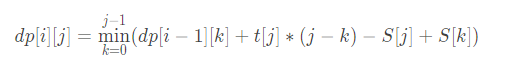# 【斜率优化】【CF311B】 Cats Transport

## 题目

Zxr960115 is owner of a large farm. He feeds mm cute cats and employs pp feeders. There’s a straight road across the farm and nn hills along the road, numbered from 1 to nn from left to right. The distance between hill ii and (i-1)(i−1) is d_{i}d
i

meters. The feeders live in hill 1.

One day, the cats went out to play. Cat ii went on a trip to hill h_{i}h
i

, finished its trip at time t_{i}t
i

, and then waited at hill h_{i}h
i

for a feeder. The feeders must take all the cats. Each feeder goes straightly from hill 1 to nn without waiting at a hill and takes all the waiting cats at each hill away. Feeders walk at a speed of 1 meter per unit time and are strong enough to take as many cats as they want.

For example, suppose we have two hills (d_{2}=1)(d
2

=1) and one cat that finished its trip at time 3 at hill 2 (h_{1}=2)(h
1

=2) . Then if the feeder leaves hill 1 at time 2 or at time 3, he can take this cat, but if he leaves hill 1 at time 1 he can’t take it. If the feeder leaves hill 1 at time 2, the cat waits him for 0 time units, if the feeder leaves hill 1 at time 3, the cat waits him for 1 time units.

Your task is to schedule the time leaving from hill 1 for each feeder so that the sum of the waiting time of all cats is minimized.

## 思路## 代码

#include<bits/stdc++.h>
#define ll long long
using namespace std;
const int N=1e5+77;
ll d[N],t[N],S[N],dp[N];
int q[N],hd[N],tl[N];

double Slope(int i,int j1,int j2)
{
return (1.0*S[j1]+dp[i][j1]-S[j2]-dp[i][j2])/(j1-j2);
}

int main(){
int n,m,p;
cin>>n>>m>>p;
if(p>=m) return puts("0"),0;
for(int i=2; i<=n; i++)
{
cin>>d[i];
d[i]+=d[i-1];
}
for(int i=1,x,p; i<=m; i++)
{
cin>>p>>x;
t[i]=-d[p]+x;
}
sort(t+1,t+1+m);
for(int i=1; i<=m; i++)
S[i]=S[i-1]+t[i];
for(int i=1; i<=m; i++)
{
for(int j=1; j<=min(i,p); j++)
{
while(hd[j-1]<tl[j-1]&&Slope(j-1,q[j-1][hd[j-1]],q[j-1][hd[j-1]+1])<=t[i])
++hd[j-1];
int tr=q[j-1][hd[j-1]];
dp[j][i]=(dp[j-1][tr]+t[i]*(i-tr)-S[i]+S[tr]);
while(hd[j]<tl[j]&&Slope(j,q[j][tl[j]],i)<Slope(j,q[j][tl[j]-1],q[j][tl[j]]))
--tl[j];
q[j][++tl[j]]=i;
}
}
cout<<dp[p][m];
}

01-3154
09-162602-08104
08-2983
09-25178
11-0932
10-0919
07-3119
08-22105